classify
Recode series into classes defined by a grid, specified limits, or quantiles.
Syntax
series_name.classify(options) spec @ outname [mapname]
Follow the classify keyword with any desired options, the “@”-sign, the name to be given the output series, and optionally the name for a valmap object describing the classification.
The form for the specification spec will depend on which of the four supported methods for classification is employed (using the “method=” option).
If the default “method=step” is employed, EViews will construct the classification using the set of intervals of size step from start through end. The spec specification is of the form
stepsize start end
where stepsize is a positive numeric value and start and end are numeric values. If start or end are explicitly set to NAs, EViews will use the corresponding minimum and maximum value of the data extended by 5% (e.g., 0.95*min or 1.05*max).
If “method=bins”, EViews will construct the classification by dividing the range between start and end into a specified number of bins. The specification is of the form:
nbins start end
where nbins in the integer number of bins. Note that depending upon whether you have selected left or right-closed intervals (using the “rightclosed” option), observations with values equal to the start or end may fall out-of-range.
Using “method=limits” specifies a classification using bins defined by a set of limit values. The spec is given by:
arg1 [arg2 arg3 ...]
where the arguments are limit values or EViews vectors containing limit values. Note that there must be at least two limit values and that the values need not be provided in ascending or descending order.
If “method=quants” is given, EViews uses the specified number of quantiles for the data, specified as an integer value. The specification is:
nquants
where nquants is the integer for the number of quantiles. For deciles you should set nquants =10, for quartiles, nquants = 4.
Options

 method=arg (default = “step”) Method for classification values: “step”– create a grid from start through end using the stepsize; “bins” – create bins by dividing the region from start to end into a specified number of bins; “quants” – create bins using the quantile values; “limits” - create bins using the specified limit points. rightclosed Bins formed using right-closed intervals.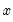is defined to be in the bin from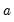to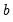if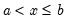. rangeerr Generate error if data value is found outside of defined bins. The default is to classify out-of-range values as NAs. q=arg (default=“r”) Quantile calculation method. “b” (Blom), “r” (Rankit-Cleveland), “o” (Ordinary), “t” (Tukey), “v” (van der Waerden), “g” (Gumbel). Only relevant where “method=quants”. encode =arg (default=“index”) Encoding method for output series: “index” – encode as integers from 0 to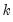whereis the number of bins, where the 0 is reserved for NA encoding if “keepna” is specified; “left” – encode using the left-most value defining the bin; “right” – encode using the right-most value defining the bin; “mid” – encode using the midpoint of the bin. keepna Classify NA values as 0 (for “encode=index” only). prompt Force the dialog to appear from within a program. p Print the results.
Examples
api5b.classify 100 200 @ api5b_ct api5b_mp
classifies the values of API5B into bins of width 100 starting at 200 and ending at the data maximum times 1.05. The classification results are saved in the series API5B_CT with associated map API5B_MP.
api5b.classify(encode=right) 100 200 1100 @ api5b_ct1
classifies API5B into bins of size 100 from 200 through 1100. The output series API5B_CT1 will have values taken from the right endpoints of the classification intervals.
api5b.classify(method=bins,rightclosed,rangeerr) 9 200 1100 @ api5b_ct2 api5b_mp2
defines 9 equally sized bins starting at 200 and ending at 1100, and classifies the data into the series API5B_CT2 with map API5B_MP2. The bins are closed on the right, and out-of-range values will generate an error.
api5b.classify(method=quants,q=g,keepna) 4 @ api5b_ct3
classifies the values of API5B into quartiles (using the Gumbel definition) in the series API5B_CT3. NA values for API5B will be encoded as 0 in the output series.
Cross-references
See “Generate by Classification” for additional discussion.